# Diffusion-Like Algorithms are Naturally Optimal''!

Introduction

For many practical applications, such as adaptive mesh based calculations, the load changes during the course of parallel computation, making it necessary to balance the load dynamicly, and in parallel.

Most of the existing parallel dynamic load balancing algorithms [11,3,10] involve two steps: flow calculation and migration. Flow calculation is to find out the amount of load to be migrated between neighboring processors, such that a uniform load distribution will be achieved when the migration is carried out to satisfy the flow. This is a well known graph theory problem with applications in many areas including parallel computing and load balancing of distributed network.

Diffusion type algorithms [1,2,9] are some of the most popular ones for flow calculations, although there are a number of other algorithms [6,2,12,13,5]. The following figure illustrates the diffusion algorithm.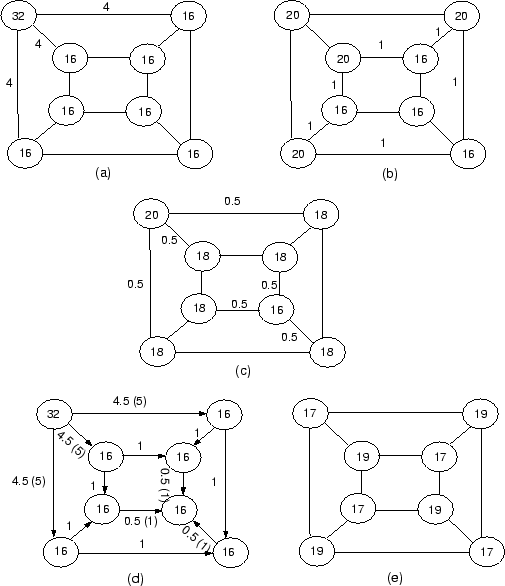The flow calculation problem usually has many solutions. To minimize the communication cost, it is important to choose a solution that involves as little load migration (flow) as possible. In , a new more efficient algorithm based on minimizing the Euclidean norm of the flow was introduced. Numerical experiment  has shown, surprisingly, that the Euclidean norm of the flow produced by the diffusion algorithm were very close to those of the new algorithm, suggesting that the diffusion algorithm also satisfies some optimal property. This conjecture has now been proved [8,7] for a general class of diffusion-like algorithms.

The Optimal Property of Diffusion-like Algorithms

The flow calculation problem is that of finding the flow along the edges of a processor graph, such that after the transfer the load of each processor will be the same. This is equivalent to solving a system of linear equations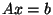with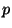equations and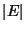unknowns (hereis the number of processors andis the number of edges in the processor graph). Because there are usually more edges than vertices in a graph, this linear system is likely to have many solutions.

The classical diffusion algorithm is really a stationary iterative algorithm: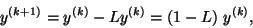(1)

where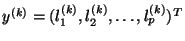is the vector of load at iteration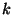,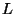is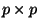the (weighted) Laplacian matrix of the processor graph.

We generalise (1) by defining the diffusion-like algorithm to be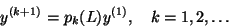(2)

where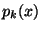is the-th order polynomial, and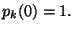By utilising this general formula, it is possible to construct diffusion-like algorithm which converges faster than the classical diffusion algorithm .

If we assume that a diffusion-like algorithm (2) converges (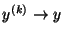), we can prove that, under mild conditions, the flow given by this diffusion-like algorithm is optimal, in the sense that it solves the following problem: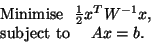(3)

Because the minimum of the optimization problem (3) is unique, this theorem suggests that the flow calculated by any diffusion algorithms of the form (2) is equivalent! Even though diffusion algorithms have this optimal property, they are not usually efficient. Effort has be made to improve the original diffusion algorithm while retaining its advantage of nearest neighbor communication [4,7]. The result of this paper reassures that the flow generated using any diffusion-like algorithms minimise the Euclidean norm of the flow.

But then the fact that diffusion-like algorithms are optimal is probably not surprising. Afterall, diffusion is a natural phenomenon, and nature always follows the path of minimal energy!

2000-03-21